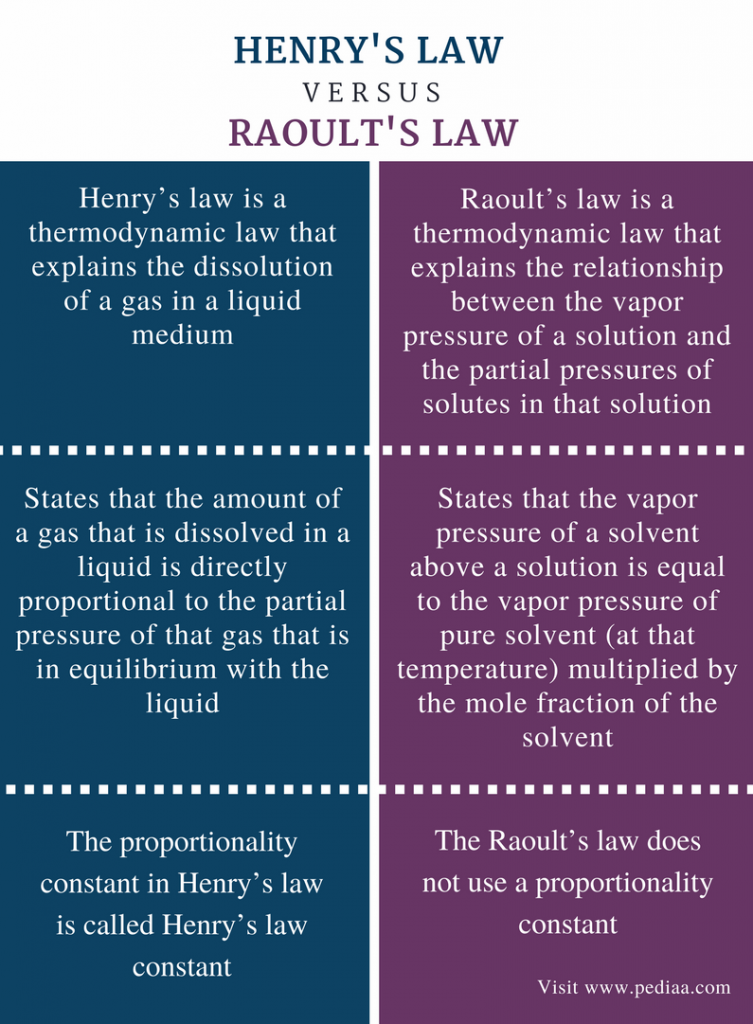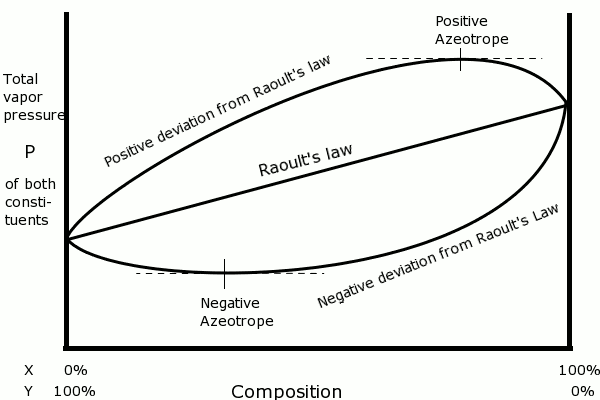# Difference Between Henry’s Law and Raoult’s Law

## Main Difference – Henry’s Law vs Raoult’s Law

Both Henry’s law and Raoult’s law are two laws found in thermodynamics and describe the relationship between a solution and its vapor that is in equilibrium with each other. Henry’s law can be used to explain the dissolution of a gas in a liquid solvent such as water. Raoult’s law indicates the behavior of solvent in a solution that is in equilibrium with its vapor pressure. However, there are certain limitations when applying these laws for real solutions. The main difference between Henry’s Law and Raoult’s Law is that Henry’s law describes the behavior of solutes of a solution whereas Raoult’s law describes the behavior of solvent in a solution.

### Key Areas Covered

1. What is Henry’s Law
– Explanation of the Law with Examples, Limitations
2. What is Raoult’s Law
– Explanation of the Law with Examples, Limitations
3. What is the Difference Between Henry’s Law and Raoult’s Law
– Comparison of Key Differences

Key Terms: Henry’s Law, Raoult’s Law, Solute, Solution, Solvent## What is Henry’s Law

Henry’s law is a gas law that explains the dissolution of a gas in a liquid medium. This law states that the amount of gas that is dissolved in a liquid is directly proportional to the partial pressure of that gas that is in equilibrium with the liquid. This can be given in an equation as below.

### [A(aq)] = kH.PA(g)

Where, [A(aq)] is the concentration of the gas A that is dissolved in the solution,

kH is Henry’s law constant

PA(g) is the partial pressure of A(g)

Henry’s law constant is a proportionality constant and is dependent on the type of solvent, solute, and the temperature. Therefore, for a particular gas, Henry’s law constant may vary at different temperatures. Therefore, when calculating the solubility of a gas in water, one should obtain the value of Henry’s law constant at that particular temperature.

 Gas Henry’s law constant at 25oC (mol/L atm) O2 1.3 x 10-3 N2 6.1 x 10-4 H2 7.8 x 10-4 CO2 3.4 x 10-2

Table 01: The Henry’s law constant at 25oC for different gasses in the atmosphere

In addition, when applying Henry’s law for a particular gas, the partial pressure should be obtained considering the vapor pressure of water at that temperature. Let’s consider the following example.

### Example

Question: Consider a lake that is in normal atmospheric conditions. Determine the solubility of O2(g) at 25oC temperature and 1atm atmospheric pressure considering the vapor pressure of water in the atmosphere as 0.0313atm. Normal air is composed of 21% of O2(g).Figure 1: A water body is composed of water with gasses dissolved in different amounts according to the temperature of the water and at atmospheric pressure.

The partial pressure of oxygen in atmosphere = (1-0.0313)atm x (21/100)
= 0.20 atm
Henry’s law constant for oxygen at 25oC          = 1.3 x 10-3mol/L atm

Applying Henry’s law;

[O2(aq)] = kH.PO2(g)
= 1.3 x 10-3 mol/L atm x 0.2 atm
= 2.6 x 10-4 mol/L

According to above calculation, the amount of dissolved oxygen in a water body at normal temperature and pressure conditions is very low.

### Limitations

Henry’s law can be used only if the molecules that are considered are in equilibrium. Moreover, this law does not work for high-pressure conditions. Moreover, if the dissolving gas shows a chemical reaction with the solvent, then this law cannot be used for that system.

## What is Raoult’s Law

Raoult’s law is a thermodynamic law that explains the relationship between the vapor pressure of a solution and the partial pressures of solutes in that solution. This law states that the vapor pressure of a solvent above a solution is equal to the vapor pressure of pure solvent (at that temperature) multiplied by the mole fraction of the solvent.
This can be given by an equation as follows.

### Psolute    =     xsolute    x    Posolute

Where, PA is the partial pressure of component A in a mixture,

xA is the mole fraction of component A,

PoA is the vapor pressure of pure component at the same temperature.

For example, let’s consider a mixture of A and B. Here,

The mole fraction of A                                                = nA/ (nA + nB)
The partial pressure of A                                            = {nA/ (nA + nB)} PoA
Therefore the total vapor pressure of that system = PA + PB

However, Raoult’s law works for only ideal solutions. Ideal solutions are composed of solutes that have intermolecular interactions between solute molecules equal to that of solvent molecules. Since there are no actual solutions that can be considered as ideal gases, we can apply this law for very dilute solutions that have a less amount of solute molecules.Figure 2: The application of Raoult’s law for a gaseous mixture composed of X and Y gases.

### Limitations

When calculating the mole fraction of a solute, one should consider the number of moles of particles present in the solution instead of the number of moles of the compound added. For example, when an ionic compound is dissolved in water, each ion that is being separated in the solution should be considered as one particle (ex: NaCl gives Na+ and Cl- ions. Thus, the amount of particles present is twice the amount of NaCl added.)

## Difference Between Henry’s Law and Raoult’s Law

### Definition

Henry’s Law: Henry’s law is a thermodynamic law that explains the dissolution of a gas in a liquid medium.

Raoult’s Law: Raoult’s law is a thermodynamic law that explains the relationship between the vapor pressure of a solution and the partial pressures of solutes in that solution.

### Concept

Henry’s Law: Henry’s law states that the amount of a gas that is dissolved in a liquid is directly proportional to the partial pressure of that gas that is in equilibrium with the liquid.

Raoult’s Law: Raoult’s law states that the vapor pressure of a solvent above a solution is equal to the vapor pressure of pure solvent (at that temperature) multiplied by the mole fraction of the solvent.

### Proportionality Constant

Henry’s Law: The proportionality constant in Henry’s law is called Henry’s law constant.

Raoult’s Law: The Raoult’s law does not use a proportionality constant.

### Conclusion

Henry’s law and Raoult’s law indicate the chemical behavior of solutions that are in contact with their vapor pressures. The difference between Henry’s law and Raoult’s law is that Henry’s law explains the behavior of solutes of a solution whereas Raoult’s law explains the behavior of solvent in a solution.

##### References:

1. “Raoult’s Law.” Chemistry LibreTexts, Libretexts, 3 Mar. 2017, Available here. Accessed 16 Aug. 2017.
2. Boundless. “Henry’s Law – Boundless Open Textbook.” Boundless, 21 Sept. 2016, Available here. Accessed 16 Aug. 2017.

##### Image Courtesy:

1. “2645374” (Public Domain) via Pixabay
2. “RaoultDeviationPressureDiagram” 由英语维基百科的Karlhahn – 從en.wikipedia轉移到共享資源。，公有领域，via Commons Wikimedia